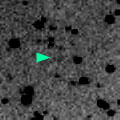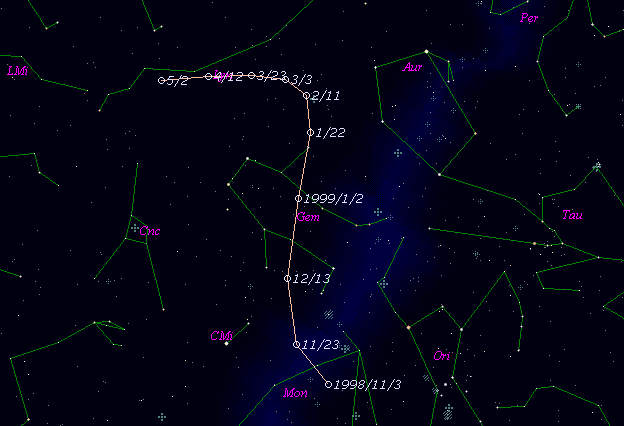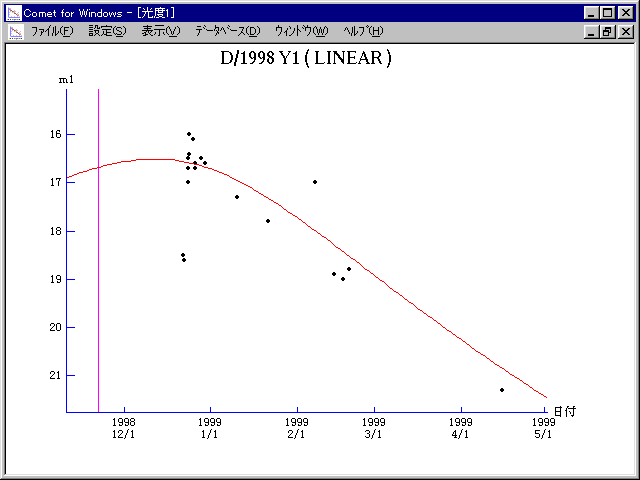# \$B%j%K%"WB@1(B

C/1998 Y1 ( LINEAR )###\$B%W%m%U%#!<%k(B

 \$BH/8+F|(B 1998\$BG/(B12\$B7n(B22\$BF|(B \$BH/8+8wEY(B 18.5\$BEy(B \$BH/8+ Lincoln Laboratory Near-Earth Asteroid Research project

###\$B###\$B50F;MWAG(B

```   The following improved orbital elements by Kenji Muraoka, are
from 117 observations 1998 Dec. 22 to 1999 Apr. 16, perturbations
by 9 Planets, Moon and 5 minor planets were taken into account.
The mean residual is +/- 0.63 arc seconds.

Epoch  =  1998 Nov.  3.0  TT       JDT = 2451120.5
T  =  1998 Nov. 21.57758       +/- 0.00084 (m.e.) TT
Peri. =  339.84128                +/- 0.00050
Node  =   98.85877                +/- 0.00062   (2000.0)
Incl. =   28.10831                +/- 0.00022
q  =    1.7469867              +/- 0.0004469 AU
e  =    0.9238893              +/- 0.0000137
a  =   22.9532341              +/- 0.0041705 AU
n  =    0.00896269             +/- 0.00000244
P  =  109.968                  +/- 0.0299710  years
(+/-11.0 days)
```

###\$B@1?^(B###\$B8wEYJQ2=(B

```        m1 = 13.2 + 5 log\$B&\$(B + 15.0 log r
```##### \$B50F;MWAG\$OB<2,7r<#;a\$N7W;;\$K\$h\$k\$b\$N\$G\$9!#(B \$B@1?^\$O(B StellaNavigator Ver.2.0 for Windows (\$B%"%9%H%m%"!<%D(B \$BJTCx(B / \$B%"%9%-!<=PHG6I4)(B) \$B\$G:n@.\$7\$?\$b\$N\$G\$9!#(B \$B8wEY%0%i%U\$O(BComet for Windows\$B\$G:n@.\$7\$?\$b\$N\$G\$9!#(B